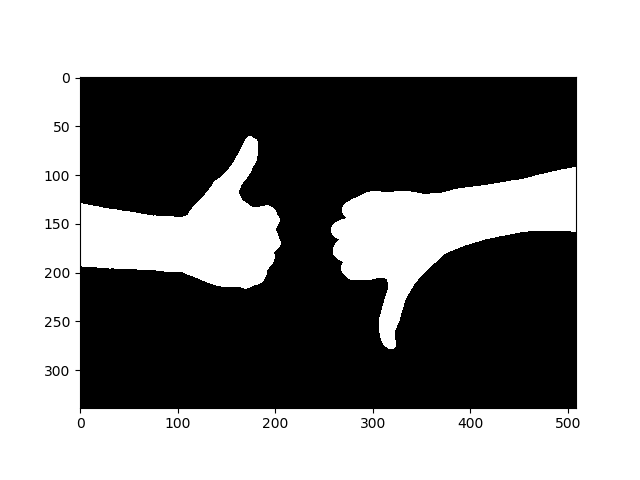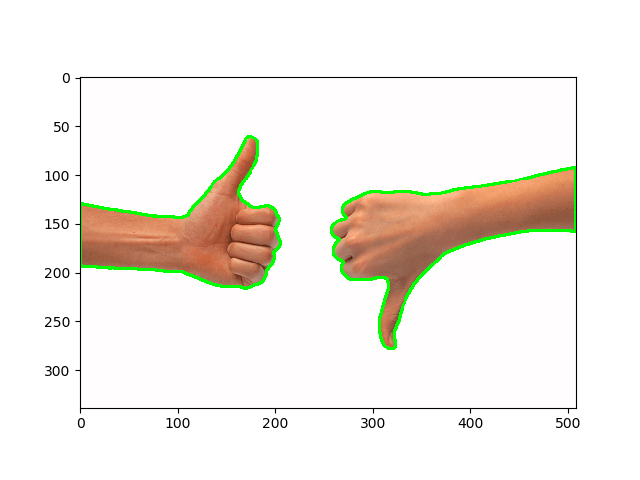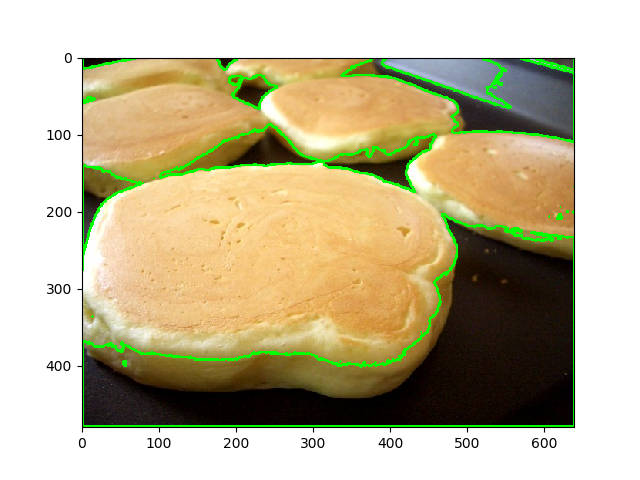Shivani Sharma — August 5, 2021

This article was published as a part of the Data Science Blogathon

The focus of Computer vision is surrounded by the extraction of meaningful information from the videos and images in computers. During this article, we’re getting to explore a great Computer Vision project using OpenCV, from beginner-level. The title for the same is ” The Edge and Contour Detection using Computer Vision”. For this project, you are going to see the description, source code, and image classification as well. So you’ll get straight to figure on this if you would like.

## Edge Detection

Edge detection is a picture processing technique for locating the boundaries of objects within images. One of the foremost popular and widely used algorithms is that the Canny edge detector. The canny edge detector is a foothold detection operator that uses a multi-stage algorithm to detect a wide range of edges in images.

The main stages are:

1. With the use of the Gaussian blur algorithm, filter out the images.

2. With the help of Sobel Filters, find out the strength and direction of edges.

3. Isolate the stronger edges and thin them to one-pixel wide lines by applying non-maximum suppression.

4. Using hysteresis to isolate the simplest edges.

Alright, let’s install OpenCV to implement it in Python:

`pip3 install opencv-python matplotlib numpy`

Open up a replacement Python file and follow along:

```import cv2
import numpy as np
import matplotlib.pyplot as plt```

Now in order to detect its edges, we have to read the images:

```# read the image

Firstly we have to convert the image to the grayscale and after that, we can proceed to bypass the image to the Canny edge detector. Let’s have a look:

```# convert it to grayscale
gray = cv2.cvtColor(image, cv2.COLOR_BGR2GRAY)```

Let’s see it:

```# show the grayscale image
plt.imshow(gray, cmap="gray")
plt.show()```With the help of the Canny algorithm, we have to mark the edges in the image that was taken as input. The input image is first delivered to the cv2.Canny() function and then used for the purpose of edge detection.

```# perform the canny edge detector to detect image edges
edges = cv2.Canny(gray, threshold1=30, threshold2=100)```

The smallest value between threshold1 and threshold2 is employed for edge linking. the most important value is employed to seek out initial segments of strong edges.

Let’s see the resulting image:

Interesting, attempt to fine-tune the edge values and see if you’ll make it better.

If you would like to use the live camera, here is that the full code for that:

```import NumPy as np
import cv2
cap = cv2.VideoCapture(0)
while True:
gray = cv2.cvtColor(frame, cv2.COLOR_BGR2GRAY)
edges = cv2.Canny(gray, 30, 100)
cv2.imshow("edges", edges)
cv2.imshow("gray", gray)
if cv2.waitKey(1) == ord("q"):
break
cap.release()
cv2.destroyAllWindows()```

The ultimate aim of detecting edges is to capture important events and perform desired changes within the properties of the planet. It’s one of the elemental steps in image processing, image pattern recognition, and computer vision techniques.

### Contour Detection

A contour can be described as a curve combining all the continual points having the same color or intensity. Basically, they show the shapes of objects contained in a picture. Contour detection may be a useful technique for shape analysis and object detection and recognition.

Contour detection isn’t the sole algorithm for image segmentation though, there are tons of others, like the present state-of-the-art semantic segmentation, hough transform, and K-Means segmentation.

For better accuracy, here is that the whole pipeline that we gonna follow to successfully detect contours in an image:

• The standardization for the input image is that it should always be in a binary form. That’s why we need to convert it to binary format.

• OpenCV function used for finding the contours is findContours().

• At last, we have to draw these contours to show the image.

Alright, let’s start. First, let’s install all the required dependencies for this project:

`pip3 install matplotlib opencv-python`

Here we are Importing the desired modules:

```import cv2
import matplotlib.pyplot as plt```

Let’s load the image used in this tutorial:

```# read the image

Converting it to RGB and then grayscale:

```# convert to RGB
image = cv2.cvtColor(image, cv2.COLOR_BGR2RGB)
# convert to grayscale
gray = cv2.cvtColor(image, cv2.COLOR_RGB2GRAY)```

As mentioned earlier during this tutorial, we gonna got to create a binary image, which suggests each pixel of the image is either black or white. You can compare it like find contours are much more similar to the detection of white objects on the blackboard. The objects which we have to find are white in color so, the boards need to be pure black.

```# create a binary thresholded image
_, binary = cv2.threshold(gray, 225, 255, cv2.THRESH_BINARY_INV)
# show it
plt.imshow(binary, cmap="gray")
plt.show()```

The binary image is created by the above code by disabling pixels i.e set it to 0 and enabling it i.i. Set it to 225. Here is that the output image:Now, this is often easy for OpenCV to detect contours:

```# find the contours from the thresholded image
contours, hierarchy = cv2.findContours(binary, cv2.RETR_TREE, cv2.CHAIN_APPROX_SIMPLE)
# draw all contours
image = cv2.drawContours(image, contours, -1, (0, 255, 0), 2)```

The above-mentioned code easily find the contours within the binary image and finally outputs them with the help of a thick Green Line to the image, let’s show it:

```# show the image with the drawn contours
plt.imshow(image)
plt.show()```#### Output image:

To achieve good results on different and world images, you would like to tune your threshold value or perform edge detection. as an example, for a pancakes image, I’ve decreased the edge to 127, here is that the result:## Difference between contour detection & edge detection:

The aim of Contour detection is surrounded by the determination of shapes of closed objects particularly because for the continual points having the same color intensity the method to hunt out the contours is to ascertain whereas edge detection is carried by detecting the change within the color intensity. Edge detection is administered for the whole image whereas contour detection is administered just for the objects within the image.

## Conclusion

In today’s race of data science, the only thing that matters is practical knowledge. The implementation of these hard and fast projects like Edge & Contour Detection gives the wings to the racer. This project is beginner-level and targets data science enthusiasts. This article aims to make you familiar with the simplest computer vision project to enhance your knowledge in Computer Vision. For any queries, you can hit my comment box. I will try my best to solve your inputs and hope to give you desired outputs. For more doubts you can input me on LinkedIn:-